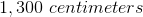# PSAT Math : Solving Word Problems with Multiple Unit Conversions

## Example Questions

### Example Question #36 : Measurement & Data

How many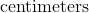are in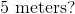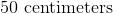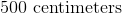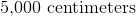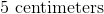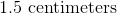Explanation:

To solve this problem we can make proportions.

We know that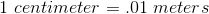and we can useas our unknown.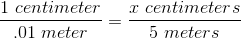Next, we want to cross multiply and divide to isolate the  on one side.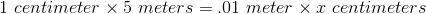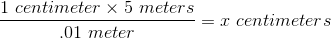The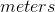will cancel and we are left with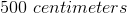### Example Question #37 : Measurement & Data

How many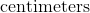are in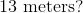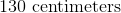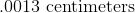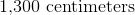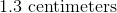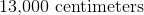Explanation:

To solve this problem we can make proportions.

We know thatand we can useas our unknown.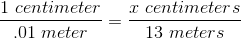Next, we want to cross multiply and divide to isolate the  on one side.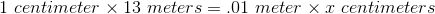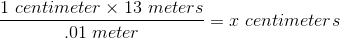Thewill cancel and we are left with